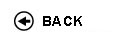Politecnico di Torino
Academic Year 2017/18
02NKIMC
Numerical and statistical methods for engineering
1st degree and Bachelor-level of the Bologna process in Civil Engineering - Torino
 Teacher Status SSD Les Ex Lab Tut Years teaching Falletta Silvia RC MAT/08 70 0 10 0 3
 SSD CFU Activities Area context MAT/07MAT/08 53 A - Di baseA - Di base Matematica, informatica e statisticaMatematica, informatica e statistica
 Subject fundamentals The course concerns the basic expertises in Scientific Calculus, Mathematical Physics and Statistics, fundamental for a professional Civil Engineer. In the Scientific Calculus part, the mathematics at the basis of the numerical methods that are widely present in the software for Engineering calculus is taught. The programming language used for the implementation of the algorithms is MATLAB. The Mathematical Physics lectures cover the main techniques that describe important physical phenomena of interest in Civil Engineering. Moreover, the mathematical models will be linked to the computational methods necessary to implement them. Finally, the Statistics lectures provide the fundamental rules of elementary probability and the models used to represent the random behavior of physical quantities in Engeneering. Expected learning outcomes solution of more complex mathematical models in Engineering, and that can not be treated with analytical methods. Mathematical Physics: the student will learn some basic techniques for the analytic resolution of differential equations with separable variables and some fundamental aspects of the finite element method for elliptic problems. All the problems considered will be of interest in Engineering and particular account will be given to heat transmission and linear elasticity. Statistics: elementary methods concerning the Mathematics of uncertainty will be aquired. The student will understand how to use some probabilistic models to deal with common problems of interest in Engineering under uncertainty or under conditions of high variability. Prerequisites / Assumed knowledge A good familiarity with the notions and the mathematical instruments introduced during the first two years of Engineering teaching is required. In particular, Linear Algebra, Integral and Differential Calculus are necessary. Moreover, knowledge of basic programming syntax is required Contents • Round up on ordinary differential equations and classical examples of ordinary differetial equations of first and second order. Partial differential equations of elliptic, parabolic and hyperbolic type (30 hours). • Generalities on numerical problems and algorithms. Short descriptions of the basic numerical methods for the resolution of linear systems, for the approximation of functions, for the numerical computation of integrals and for the resolution of ordinary differential equations (30 hours). • Elementary probability: axioms, conditional probability, Bayes' theorem. The use of continuous distributions, such as the normal (gaussian) and the log-normal, to represent the random behavior of physical engineering quantities. Elements for the simulation of random systems (20 hours). Delivery modes Each of the above mentioned arguments will be developed both with theoretical lectures and exercises. Both will be delivered partially in the lecture room and partially in the computer room. In particular, seven computer lectures (10 hours) concerning the scientific calculus will be delivered in the informatic laboratory where, by using the MATLAB software, the students will implement the algorithms described in the lectures. Texts, readings, handouts and other learning resources • T. J. R. Hughes, "The Finite Element Method: Linear Static and Dynamic Finite Element Analysis", Dover Publ. Inc., 2000. • G. Monegato, "Metodi e algoritmi per il Calcolo Numerico", CLUT, 2008. • L. Scuderi, "Laboratorio di Calcolo Numerico", CLUT, 2005. • A. N. Tichonov, A.A Samarskij, "Equazioni della fisica matematica", edizioni MIR, 1981. • S.M. Ross, "Probability and Statistics for Engineering and the Sciences", Academic Press.. Further course material such as lectures on-line, lecture notes, proposed and solved exercises will be given through the ‘portale della didattica’ website Assessment and grading criteria The final exam consists of a two hours written test and, upon request by the teachers, of an oral exam. The exam includes exercises and/or theoretical questions. The minimum mark to pass the written exam is 18; at least 5 points must come from exercises of Mathematical Physics, at least 5 points must come from exercises of Scientific Calculus and at least 4 points from exercises of Statistics. During the exam, no course material is allowed. It is forbidden to use electronic devices, mobile phones, scientific calculators, books and notes. Programma definitivo per l'A.A.2017/18© Politecnico di Torino
Corso Duca degli Abruzzi, 24 - 10129 Torino, ITALY